| 会员服务 | 登录 | 注册 |

|

|

| 消息 | 帮助中心 | 服务热线 : 400-085-2125• ￥2
• ￥5
• ￥10
• ￥50
• ￥100
• ￥200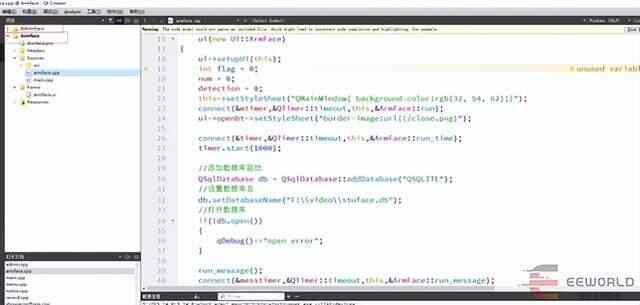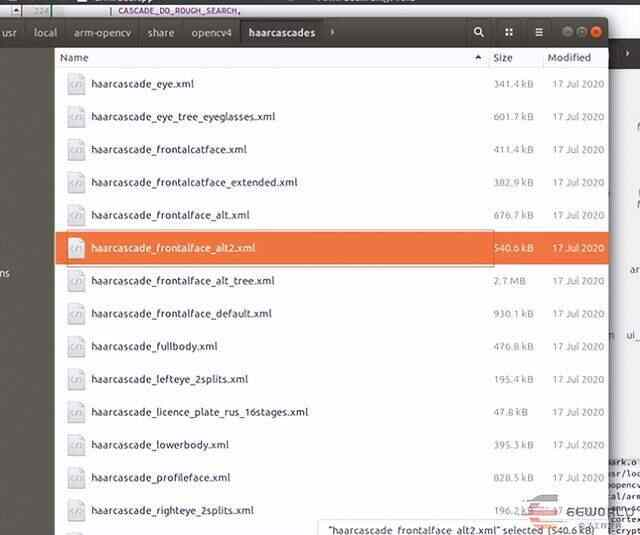OpenCV编译完成后已经提供好了的。

//将opencv官方训练好的人脸识别分类器拷贝到自己的工程目录中

VideoCapture cap(1); //0表示电脑自带的，如果用一个外接摄像头，将0变成1

Mat frame, myFace;

int pic_num = 1;

while (1) {

//摄像头读图像

cap >> frame;

vector faces;//vector容器存检测到的faces

Mat frame_gray;

cvtColor(frame, frame_gray, COLOR_BGR2GRAY);//转灰度化，减少运算

cascada.detectMultiScale(frame_gray, faces, 1.1, 4, CV_HAAR_DO_ROUGH_SEARCH, Size(70, 70), Size(1000, 1000));

printf("检测到人脸个数：%dn", faces.size());

//识别到的脸用矩形圈出

for (int i = 0; i < faces.size(); i++)

{

rectangle(frame, faces, Scalar(255, 0, 0), 2, 8, 0);

}

//当只有一个人脸时，开始拍照

if (faces.size() == 1)

{

Mat faceROI = frame_gray(faces);//在灰度图中将圈出的脸所在区域裁剪出

//cout << faces.x << endl;//测试下face.x

resize(faceROI, myFace, Size(92, 112));//将兴趣域size为92*112

putText(frame, to_string(pic_num), faces.tl(), 3, 1.2, (0, 0, 225), 2, 0);//在 faces.tl()的左上角上面写序号

string filename = format("F:video%d.jpg", pic_num); //图片的存放位置，frmat的用法跟QString差不多

imwrite(filename, myFace);//存在当前目录下

imshow(filename, myFace);//显示下size后的脸

waitKey(500);//等待500us

destroyWindow(filename);//:销毁指定的窗口

pic_num++;//序号加1

if (pic_num == 11)

{

return 0;//当序号为11时退出循环,一共拍10张照片

}

}

int c = waitKey(10);

if ((char)c == 27) { break; } //10us内输入esc则退出循环

imshow("frame", frame);//显示视频流

waitKey(100);//等待100us

}

return 0;

//读取你的CSV文件路径.

//string fn_csv = string(argv);

string fn_csv = "F:videocccat.txt";

// 2个容器来存放图像数据和对应的标签

vector images;

vector labels;

// 读取数据. 如果文件不合法就会出错

// 输入的文件名已经有了.

try

{

read_csv(fn_csv, images, labels); //从csv文件中批量读取训练数据

}

catch (cv::Exception& e)

{

cerr << "Error opening file "" << fn_csv << "". Reason: " << e.msg << endl;

// 文件有问题，我们啥也做不了了，退出了

exit(1);

}

// 如果没有读取到足够图片，也退出.

if (images.size() <= 1) {

string error_message = "This demo needs at least 2 images to work. Please add more images to your data set!";

CV_Error(CV_StsError, error_message);

}

for (int i = 0; i < images.size(); i++)

{

//cout<

if (images.size() != Size(92, 112))

{

cout << i << endl;

cout << images.size() << endl;

}

}

// 下面的几行代码仅仅是从你的数据集中移除最后一张图片，作为测试图片

//[gm:自然这里需要根据自己的需要修改，他这里简化了很多问题]

Mat testSample = images[images.size() - 1];

int testLabel = labels[labels.size() - 1];

images.pop_back();//删除最后一张照片，此照片作为测试图片

labels.pop_back();//删除最有一张照片的labels

// 下面几行创建了一个特征脸模型用于人脸识别，

// 通过CSV文件读取的图像和标签训练它。

// T这里是一个完整的PCA变换

//如果你只想保留10个主成分，使用如下代码

// cv::createEigenFaceRecognizer(10);

//

// 如果你还希望使用置信度阈值来初始化，使用以下语句：

// cv::createEigenFaceRecognizer(10, 123.0);

//

// 如果你使用所有特征并且使用一个阈值，使用以下语句：

// cv::createEigenFaceRecognizer(0, 123.0);

//创建一个PCA人脸分类器，暂时命名为model吧，创建完成后

//调用其中的成员函数train()来完成分类器的训练

Ptr model = face::EigenFaceRecognizer::create();

model->train(images, labels);

model->save("MyFacePCAModel.xml");//保存路径可自己设置，但注意用“”

Ptr model1 = face::FisherFaceRecognizer::create();

model1->train(images, labels);

model1->save("MyFaceFisherModel.xml");

Ptr model2 = face::LBPHFaceRecognizer::create();

model2->train(images, labels);

model2->save("MyFaceLBPHModel.xml");

// 下面对测试图像进行预测，predictedLabel是预测标签结果

//注意predict()入口参数必须为单通道灰度图像，如果图像类型不符，需要先进行转换

//predict()函数返回一个整形变量作为识别标签

int predictedLabel = model->predict(testSample);//加载分类器

int predictedLabel1 = model1->predict(testSample);

int predictedLabel2 = model2->predict(testSample);

// 还有一种调用方式，可以获取结果同时得到阈值:

// int predictedLabel = -1;

// double confidence = 0.0;

// model->predict(testSample, predictedLabel, confidence);

string result_message = format("Predicted class = %d / Actual class = %d.", predictedLabel, testLabel);

string result_message1 = format("Predicted class = %d / Actual class = %d.", predictedLabel1, testLabel);

string result_message2 = format("Predicted class = %d / Actual class = %d.", predictedLabel2, testLabel);

cout << result_message << endl;

cout << result_message1 << endl;

cout << result_message2 << endl;

getchar();

//waitKey(0);

return 0;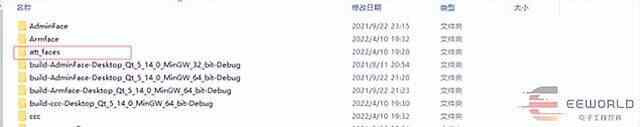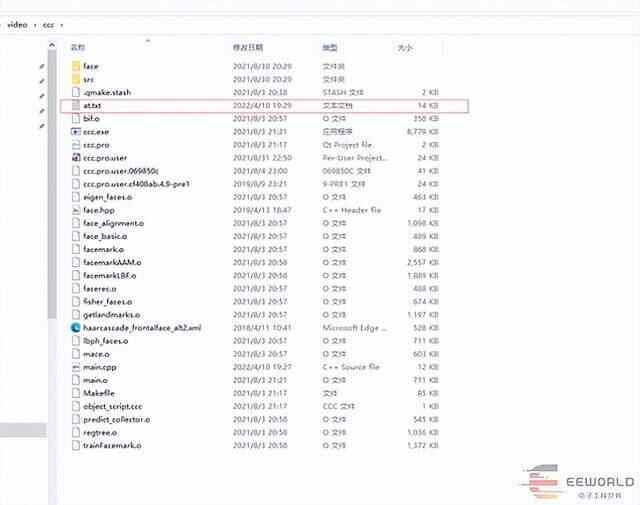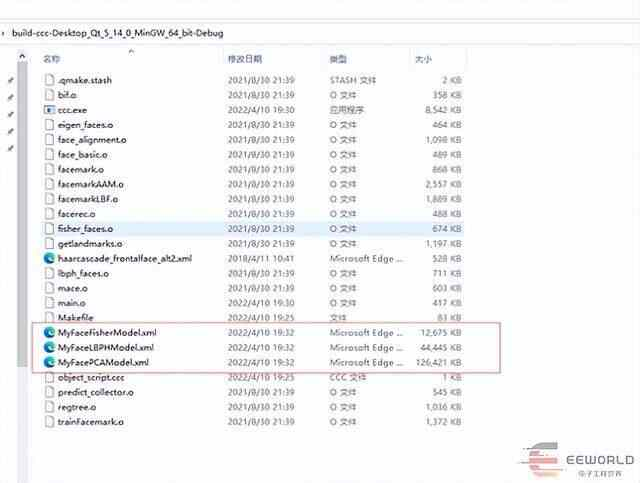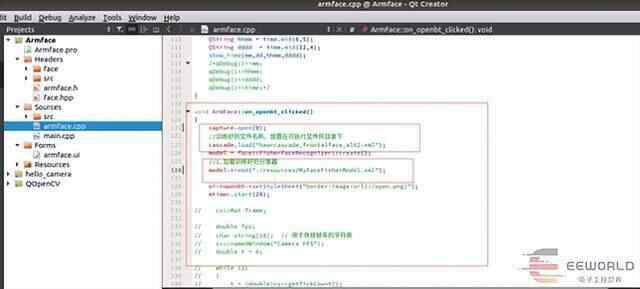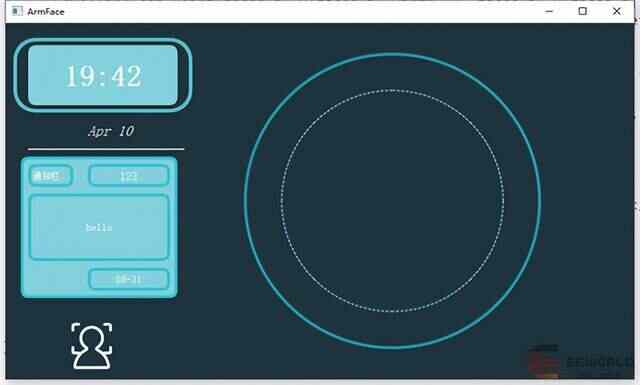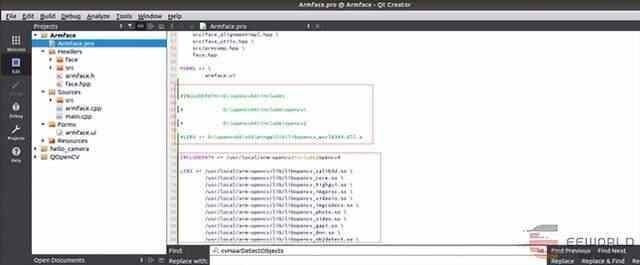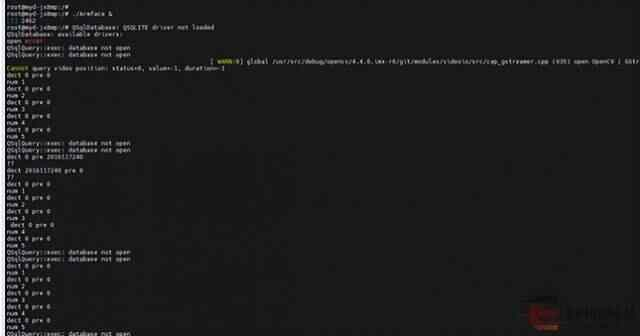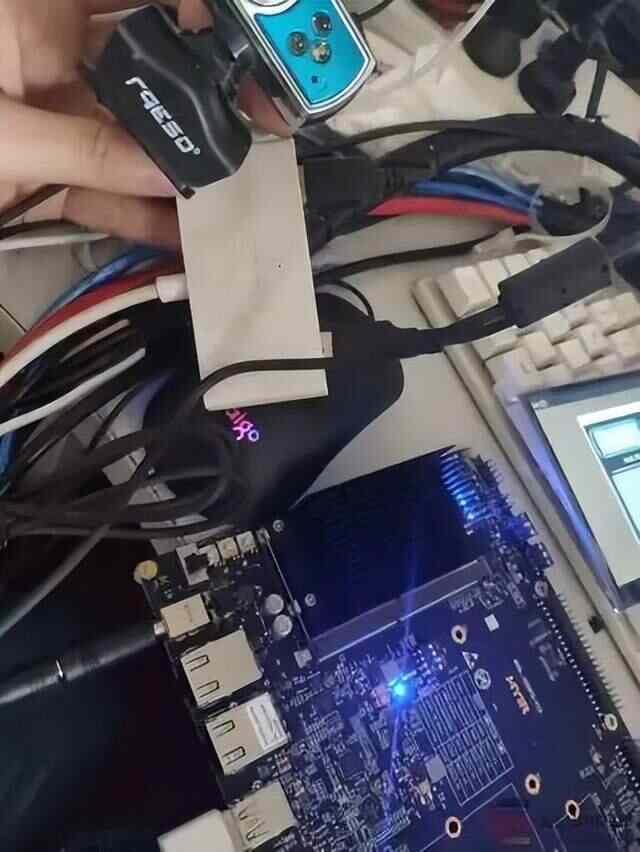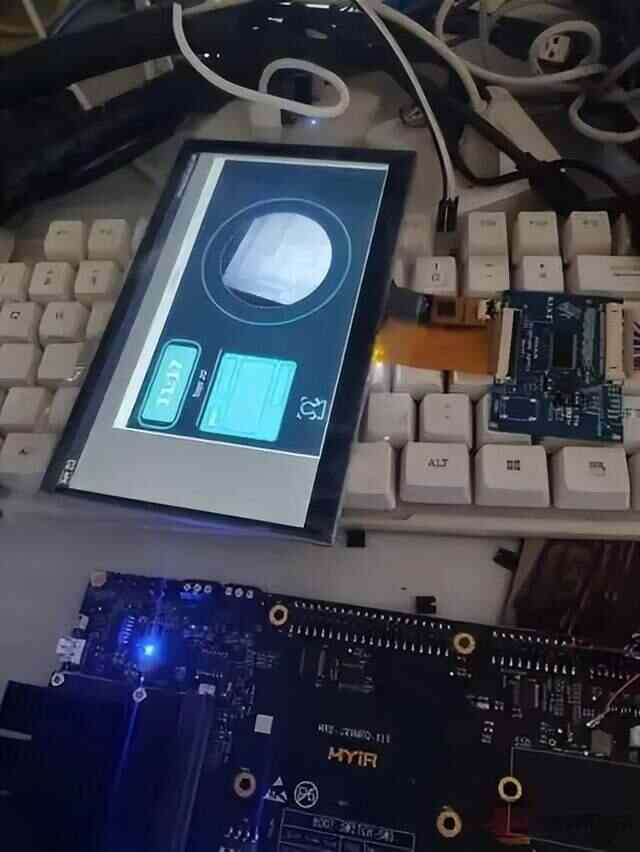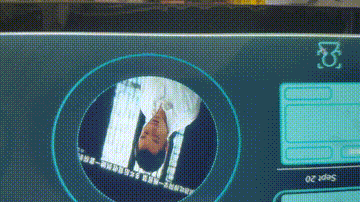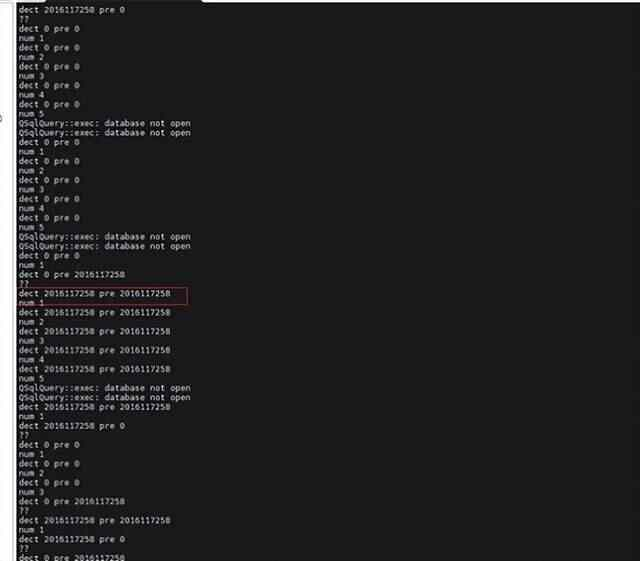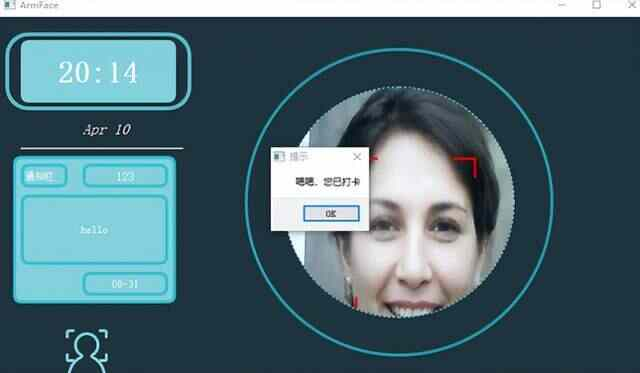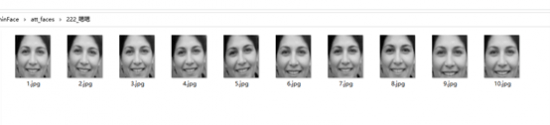http://www.myir-tech.com/product/myd-jx8mpq.htm

• 联系方式:
400-085-2125
• 关注公众号
随时随地发包接包，
还能查看项目进度